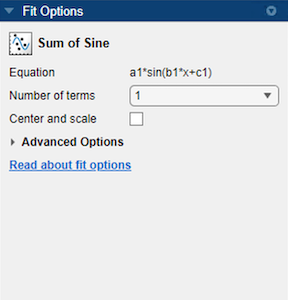## Sum of Sines Models

### About Sum of Sines Models

The sum of sines model fits periodic functions, and is given by

`$y=\sum _{i=1}^{n}{a}_{i}\mathrm{sin}\left({b}_{i}x+{c}_{i}\right)$`

where a is the amplitude, b is the frequency, and c is the phase constant for each sine wave term. n is the number of terms in the series and 1 ≤ n ≤ 8. This equation is closely related to the Fourier series described in Fit Fourier Models. The main difference is that the sum of sines equation includes the phase constant, and does not include a constant (intercept) term.

### Fit Sum of Sine Models Interactively

1. Open the Curve Fitter app by entering `curveFitter` at the MATLAB® command line. Alternatively, on the Apps tab, in the Math, Statistics and Optimization group, click Curve Fitter.

2. In the Curve Fitter app, select curve data. On the Curve Fitter tab, in the Data section, click Select Data. In the Select Fitting Data dialog box, select X data and Y data, or just Y data against an index.

3. Click the arrow in the Fit Type section to open the gallery, and click Sum of Sine in the Regression Models group.You can specify the following options in Fit Options pane:

• Specify the number of terms as a positive integer in the range [1 8]. Look in the Results pane to see the model terms, values of the coefficients, and goodness-of-fit statistics.

• Optionally, in the Advanced Options section, specify coefficient starting values and constraint bounds, or change algorithm settings. The app calculates optimized start points for Sum of Sine fits, based on the data set. You can override the start points and specify your own values in the Fit Options pane.

The Sum of Sine fit has a lower bound constraint on bi of `0`. The default lower bounds for most fits in the Fit Type section are `-Inf`.

For more information on the settings, see Specify Fit Options and Optimized Starting Points.

### Selecting a Sum of Sine Fit at the Command Line

Specify the model type `sin` followed by the number of terms, e.g., `'sin1'` to `'sin8'`.

For example, to load some periodic data and fit a six-term sum of sine model:

```load enso; f = fit( month, pressure, 'sin6') plot(f,month,pressure)```

If you want to modify fit options such as coefficient starting values and constraint bounds appropriate for your data, or change algorithm settings, see the table of additional properties with `NonlinearLeastSquares` on the `fitoptions` reference page.